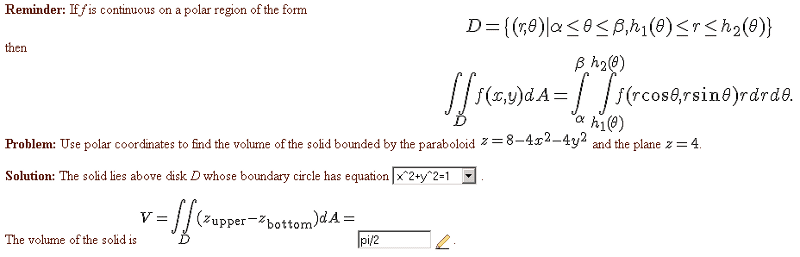# Using polar coordinates to find the volume of a bounded solid

Using polar coordinates to find the volume of a bounded solid[Solved]I found the equation of the boundary circle by setting z to 4 in the paraboloid.
Then I did some work to get polar coords:
$$x^2+y^2 = 1$$
$$x^2+y^2 = r^2$$
$$1-x^2-y^2 = 1-r^2$$
Then I set up my integral as such
$$\int_0^{2\pi}\int_{0}^{1}(1-r^2)rdrd\theta$$
After the double integration, I get pi/2.

edit: It should be 4r-4r3 as the integrand.

Last edited:

vela
Staff Emeritus
Homework Helper